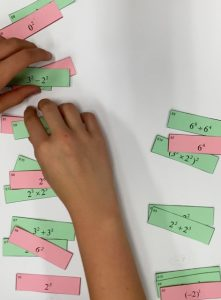Working either in small groups or as a whole-class activity, you will be given two sets of small cards. On one set expressions, such as 68 ÷ 64, are given. In the other set single values, such as 64, are given. Work out the value of the cards using the laws of exponents or by calculation, and put cards with the same value in groups. Each group should have AT LEAST one card of each colour (use the blank card where necessary). You should end up with ten groups of cards.

Whilst doing the activity, try and think about why exponent rules work. Answer some of these questions:

What is the difference between 52 and 5×2? What does 56 mean? If I divide this by 5, what is the result? How would you write this as a power of 5?

What is the answer to 23 – 22? How would you write this as an integer? How would you write this as a power of 2? Why should you not subtract the powers?

Note: This activity was designed as a formative assessment activity, in order to to provide the teacher with information about the learners’ current levels of understanding.

Exponents Card Set

Guidance (for teachers)

Tagged with: